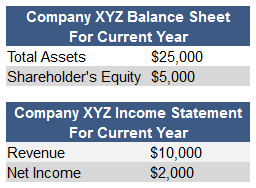## What is DuPont Analysis?

DuPont analysis examines the return on equity (ROE) analyzing profit margin, total asset turnover, and financial leverage. It was created by the DuPont Corporation in the 1920s.

## How Does DuPont Analysis Work?

The DuPont analysis is also referred to as the DuPont identity.

In a DuPont analysis, the formula for ROE is:

ROE = Profit Margin x Total Asset Turnover x Leverage factor

The formula breaks down further to:

ROE = (Net Income/Revenues) x (Revenues/Total Assets) x (Total Assets/ Shareholders' Equity)

For example, let's consider the following information for Company XYZ:Using the formula above, we can calculate that Company XYZ's ROE is:

ROE = (\$2,000/\$10,000) x (\$10,000/\$25,000) x (\$25,000/\$5,000) = 0.20 x 0.40 x 5 = 0.40 or 40%

The DuPont analysis analyzes the numbers shown in profit margin (\$2,000/\$10,000), total asset turnover (\$10,000/\$25,000) and leverage factor (\$25,000/\$5,000) to find Company XYZ's ROE.

## Why Does DuPont Analysis Matter?

The DuPont Analysis is important determines what is driving a company's ROE; Profit margin shows the operating efficiency, asset turnover shows the asset use efficiency, and leverage factor shows how much leverage is being used.

The method goes beyond profit margin to understand how efficiently a company's assets generate sales or cash and how well a company uses debt to produce incremental returns.

Using these three factors, a DuPont analysis allows analysts to dissect a company, efficiently determine where the company is weak and strong and quickly know what areas of the business to look at (i.e., inventory management, debt structure, margins) for more answers. The measure is still broad, however, and is not a substitute for detailed analysis.

The DuPont analysis looks uses both the income statement as well as the balance sheet to perform the examination. As a result, major asset purchases, acquisitions, or other significant changes can distort the ROE calculation. Many analysts use average assets and shareholders' equity to mitigate this distortion, although that approach assumes the balance sheet changes occurred steadily over the course of the year, which may not be accurate either.